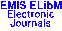PUBLICATIONS DE L'INSTITUT MATHÉMATIQUE (BEOGRAD) (N.S.) Vol. 70(84), pp. 69--78 (2001)

Previous Article

Next Article

Contents of this Issue

Other Issues

ELibM Journals

ELibM Home

EMIS Home

## ON THE CONVERGENCE OF FINITE-DIFFERENCE SCHEME FOR ONE NONLOCAL ELLIPTIC BOUNDARY VALUE PROBLEM

### Givi Berikelashvili

Abstract: The finite-difference scheme approximating nonlocal boundary value problem for a second order elliptic equation is studied. A convergence rate estimate in discrete \$W_2^1\$-norm is obtained, assuming that the coefficients and the solution to the original problem belongs to Sobolev spaces.

Keywords: difference schemes; nonlocal boundary value problem; elliptic equation; weighted spaces; convergence rate

Classification (MSC2000): 65N06

Full text of the article: